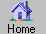Prev Next Index-> contents reference index search external Up-> CppAD Example get_started.cpp CppAD-> Install Introduction AD ADFun preprocessor multi_thread utility ipopt_solve Example speed Appendix Example-> get_started.cpp General ExampleUtility ListAllExamples testvector wno_conversion get_started.cpp Headings-> Purpose Function Derivative Value Poly Exercises Program Output Running

Getting Started Using CppAD to Compute Derivatives

Purpose
Demonstrate the use of CppAD by computing the derivative of a simple example function.

Function
The example function $\mathit{f}:\mathbf{R}\to \mathbf{R}$ is defined by $\mathit{f}\left(\mathit{x}\right)={\mathit{a}}_{0}+{\mathit{a}}_{1}*{\mathit{x}}^{1}+\cdots +{\mathit{a}}_{\mathit{k}-1}*{\mathit{x}}^{\mathit{k}-1}$ where `a` is a fixed vector of length `k` .

Derivative
The derivative of $\mathit{f}\left(\mathit{x}\right)$ is given by $\mathit{f}\text{'}\left(\mathit{x}\right)={\mathit{a}}_{1}+2*{\mathit{a}}_{2}*\mathit{x}+\cdots +\left(\mathit{k}-1\right)*{\mathit{a}}_{\mathit{k}-1}*{\mathit{x}}^{\mathit{k}-2}$
Value
For the particular case in this example, $\mathit{k}$ is equal to 5, $\mathit{a}=\left(1,1,1,1,1\right)$ , and $\mathit{x}=3$ . If follows that $\mathit{f}\text{'}\left(3\right)=1+2*3+3*{3}^{2}+4*{3}^{3}=142$
Poly
The routine `Poly` is defined below for this particular application. A general purpose polynomial evaluation routine is documented and distributed with CppAD (see Poly ).

Exercises
1. Compute and print the derivative of $\mathit{f}\left(\mathit{x}\right)=1+\mathit{x}+{\mathit{x}}^{2}+{\mathit{x}}^{3}+{\mathit{x}}^{4}$ at the point $\mathit{x}=2$ .
2. Compute and print the derivative of $\mathit{f}\left(\mathit{x}\right)=1+\mathit{x}+{\mathit{x}}^{2}/2$ at the point $\mathit{x}=.5$ .
3. Compute and print the derivative of $\mathit{f}\left(\mathit{x}\right)=\mathrm{exp}\left(\mathit{x}\right)-1-\mathit{x}-{\mathit{x}}^{2}/2$ at the point $\mathit{x}=.5$ .

Program
```#include <iostream>      // standard input/output
#include <vector>        // standard vector

namespace {
// define y(x) = Poly(a, x) in the empty namespace
template <class Type>
Type Poly(const std::vector<double> &a, const Type &x)
{     size_t k  = a.size();
Type y   = 0.;  // initialize summation
Type x_i = 1.;  // initialize x^i
size_t i;
for(i = 0; i < k; i++)
{     y   += a[i] * x_i;  // y   = y + a_i * x^i
x_i *= x;           // x_i = x_i * x
}
return y;
}
}
// main program
int main(void)
using std::vector;         // use vector as abbreviation for std::vector
size_t i;                  // a temporary index

// vector of polynomial coefficients
size_t k = 5;              // number of polynomial coefficients
vector<double> a(k);       // vector of polynomial coefficients
for(i = 0; i < k; i++)
a[i] = 1.;           // value of polynomial coefficients

// domain space vector
size_t n = 1;              // number of domain space variables
vector< AD<double> > X(n); // vector of domain space variables
X = 3.;                 // value corresponding to operation sequence

// declare independent variables and start recording operation sequence

// range space vector
size_t m = 1;              // number of ranges space variables
vector< AD<double> > Y(m); // vector of ranges space variables
Y = Poly(a, X);      // value during recording of operations

// store operation sequence in f: X -> Y and stop recording

// compute derivative using operation sequence stored in f
vector<double> jac(m * n); // Jacobian of f (m by n matrix)
vector<double> x(n);       // domain space vector
x = 3.;                 // argument value for derivative
jac  = f.Jacobian(x);      // Jacobian for operation sequence

// print the results
std::cout << "f'(3) computed by CppAD = " << jac << std::endl;

// check if the derivative is correct
int error_code;
if( jac == 142. )
error_code = 0;      // return code for correct case
else  error_code = 1;      // return code for incorrect case

return error_code;
}```

Output
Executing the program above will generate the following output: ``` f'(3) computed by CppAD = 142 ```
Running
To build and run this program see cmake_check .
Input File: example/get_started/get_started.cpp